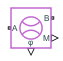# Mass & Energy Flow Rate Sensor (G)

Measure mass and energy flow rates

• Library:
• Simscape / Foundation Library / Gas / Sensors

•## Description

The Mass & Energy Flow Rate Sensor (G) block represents an ideal sensor that measures mass flow rate and energy flow rate in a gas network. The energy flow rate is the advection of total enthalpy. There is no change in pressure or temperature across the sensor.

Because the flow rates are Through variables, the block must connect in series with the component being measured.

The relative orientation of ports A and B establishes the measurement sign. The sign is positive if flow occurs from port A to port B. Switching port connections reverses the measurement sign.

Two physical signal ports output the measurement data. Port M outputs the mass flow rate. Port Phi outputs the energy flow rate. Connect the ports to PS-Simulink Converter blocks to transform the output physical signals into Simulink® signals, for example, for plotting or additional data processing.

## Ports

### Output

expand all

Physical signal output port for mass flow rate measurement.

Physical signal output port for energy flow rate measurement.

### Conserving

expand all

Gas conserving port. Mass and energy flow rates are positive if gas flows from port A to port B.

Gas conserving port. Mass and energy flow rates are positive if gas flows from port A to port B.

## Version History

Introduced in R2016b